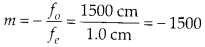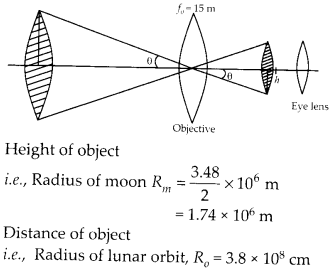Enlightened

# Question 14: NCERT Solutions for 12th Class Physics: Chapter 9-Ray Optics and Optical Instruments

• 0

Question 14: NCERT Solutions for 12th Class Physics: Chapter 9-Ray Optics and Optical Instruments

(a) A giant refracting telescope at an observatory has an objective lens of focal length 15 m. If an eyepiece of focal length 1.0 cm is used, what is the angular magnification of the telescope?
(b) If this telescope is used to view the moon, what is the diameter of the image of the moon formed by the objective lens? The diameter of the moon is 3.48 × 106 m and the radius of lunar orbit is 3.8 × 108 sm.

Share

1. Solution:
(a) ƒo = 15 m and ƒe = 1.0 cm angular magnification by the telescope normal adjustment(b) The image of the moon by the objective at lens is formed on its focus only as the moon is nearly infinite distance as compared to focal length.Distance of object i.e., Radius of lunar orbit, Ro = 3.8 × 108 cm Distance of image for objective lens i.e., focal length of objective lens ƒo = 15 m Radius of image of moon by objective lens can be calculated.Check the complete chapter with solutions.

NCERT Solutions for 12th Class Physics: Chapter 9-Ray Optics and Optical Instruments

• 0